## Calculations with Equilibrium Constants

### Learning Objectives

• Perform equilibrium constant calculations.

### Iron-poor blood?Iron is an important component of red cells. Patients who have low iron will usually be anemic and have a lower than normal number of red blood cells. One way to assess serum iron concentration is with the use of Ferrozine, a complex organic molecule. Ferrozine forms a product with Fe 3+ , producing a pink color. In order to determine factors affecting the reaction, we need to measure the equilibrium constant. If the equilibrium does not lie far in the direction of products, precautions need to be taken when using this material to measure iron in serum.

## Calculations with Equilibrium Constants

The general value of the equilibrium constant gives us information about whether the reactants or the products are favored at equilibrium. Since the product concentrations are in the numerator of the equilibrium expression, a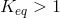means that the products are favored over the reactants. A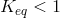means that the reactants are favored over the products.

Though it would often seem that the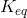value would have various units depending on the values of the exponents in the expression, the general rule is that any units are dropped. Allvalues will be reported as having no units.

### Sample Problem: Calculating an Equilibrium Constant

Equilibrium occurs when nitrogen monoxide gas reacts with oxygen gas to form nitrogen dioxide gas.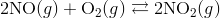At equilibrium at 230°C, the concentrations are measured to be [NO] = 0.0542 M, [O 2 ] = 0.127 M, and [NO 2 ] = 15.5 M. Calculate the equilibrium constant at this temperature.

Step 1: List the known values and plan the problem .

Known

• [NO] = 0.0542 M
• [O 2 ] = 0.127 M
• [NO 2 ] = 15.5 M

Unknown

•value

The equilibrium expression is first written according to the general form in the text. The equilibrium values are substituted into the expression and the value calculated.

Step 2: Solve .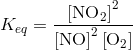Substituting in the concentrations at equilibrium: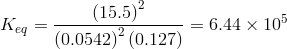The equilibrium concentration of the product NO 2 is significantly higher than the concentrations of the reactants NO and O 2 . As a result, thevalue is much larger than 1, an indication that the product is favored at equilibrium.

The equilibrium expression only shows those substances whose concentrations are variable during the reaction. A pure solid or a pure liquid does not have a concentration that will vary during a reaction. Therefore, an equilibrium expression omits pure solids and liquids and only shows the concentrations of gases and aqueous solutions. The decomposition of mercury(II) oxide can be shown by the following equation, followed by its equilibrium expression.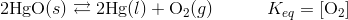The stoichiometry of an equation can also be used in a calculation of an equilibrium constant. At 40°C, solid ammonium carbamate decomposes to ammonia and carbon dioxide gases.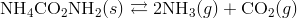At equilibrium, the [CO 2 ] is found to be 4.71 × 10 -3 M. Can thevalue be calculated from just that information? Because the ammonium carbamate is a solid, it is not present in the equilibrium expression.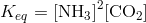The stoichiometry of the chemical equation indicates that as the ammonium carbamate decomposes, 2 mol of ammonia gas is produced for every 1 mol of carbon dioxide. Therefore, at equilibrium, the concentration of the ammonia will be twice the concentration of carbon dioxide. So [NH 3 ] = 2 × (4.71 × 10 -3 ) = 9.42 × 10 -3 M. Substituting these values into theexpression: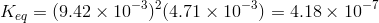### Using Equilibrium Constants

The equilibrium constants are known for a great many reactions. Hydrogen and bromine gases combine to form hydrogen bromide gas. At 730°C, the equation andare given below.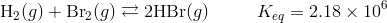A certain reaction is begun with only HBr. When the reaction mixture reaches equilibrium at 730°C, the concentration of bromine gas is measured to be 0.00243 M. What is the concentration of the H 2 and the HBr at equilibrium?

Since the reaction begins with only HBr and the mole ratio of H 2 to Br 2 is 1:1, the concentration of H 2 at equilibrium is also 0.00243 M. The equilibrium expression can be rearranged to solve for the concentration of HBr at equilibrium.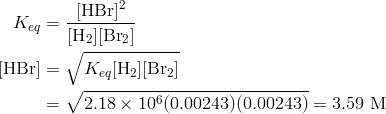Since the value of the equilibrium constant is very high, the concentration of HBr is much greater than that of H 2 and Br 2 at equilibrium.

### Summary

• Calculation of an equilibrium constant is described.

### Practice

Work problems 1-4 at ChemTopics.com.

### Review

1. What are the units for?
2. Why is the temperature specified in equilibrium problems?
3. Why don’t we include solids or liquids in equilibrium calculations?